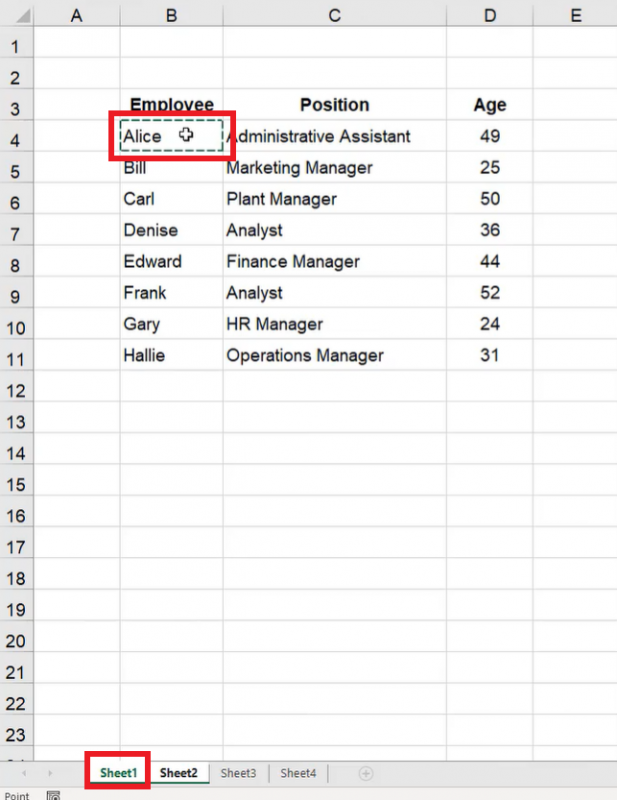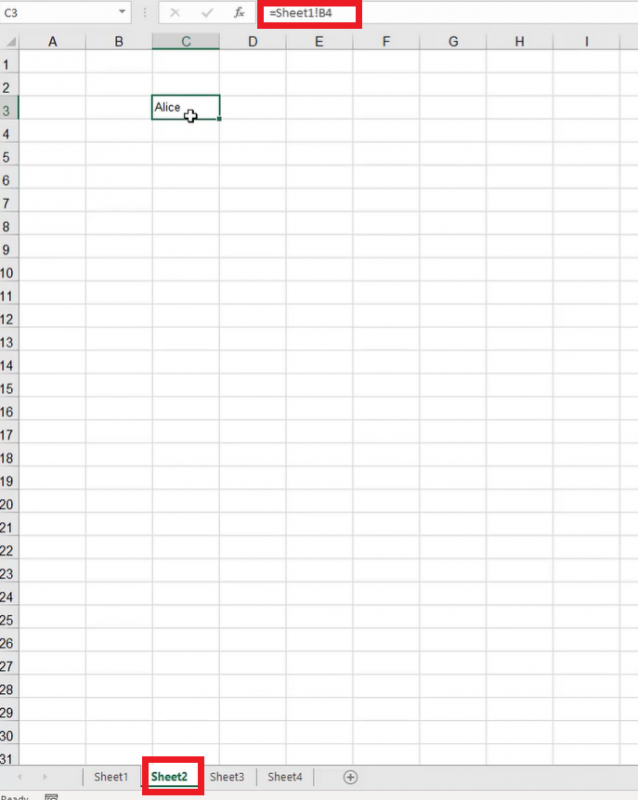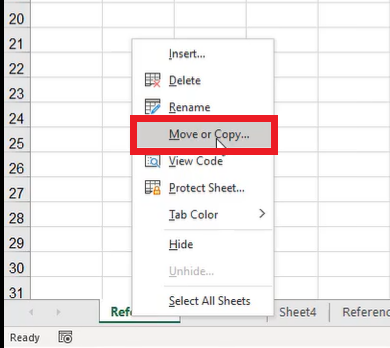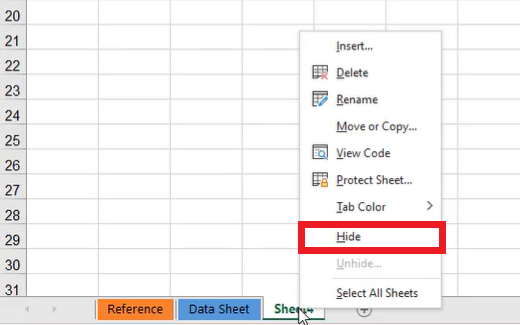# Managing Worksheets

This chapter covers referencing and managing worksheets in Excel.

Use this workbook for the chapter.

## Workbook vs. Worksheet

There is a difference between workbooks and worksheets in Excel. When you open up a spreadsheet, save it, and give it a name, you have created a workbook. A workbook can contain one or more worksheets, which can be found at the bottom of the page (See Figure 5.1). The smallest reference point in a workbook is an individual cell. A page full of cells is called a worksheet. A file full of worksheets is called a workbook.

To add more worksheets, select the plus sign in the bottom left of the screen (see Figure 5.2). A new worksheet will open, and a new tab will appear at the bottom. You can use this step to make as many worksheets within the workbook as you need.

## Referencing Cells Across Worksheets

Cells in each worksheet can be referenced across worksheets. To do this, use the following steps:

1. Select a cell, and type the equals symbol, =.
2. Select the desired worksheet.
3. Select the cell you want to input into the cell on the first worksheet. (See Figure 5.3)Figure 5.3

1. Excel will bring that value over to the second worksheet. (See Figure 5.4)
2. The formula bar will read Sheet1!B4.
1. If the worksheet you are referencing has a different name, the formula bar will have the name of the worksheet, an exclamation point, and the cell number that the original value is found in.Figure 5.4

Selecting the cell and dragging down or to the right will bring over more information from the other worksheet (see Figure 5.5 and Figure 5.6), just like you learned in the Absolute and Relative Referencing chapter.

## Making an Absolute Reference

If you don’t want the rest of the data from the other worksheet to appear when you drag down or to the right, you need to anchor the desired data by making it an absolute reference.

To make an absolute reference, use the following steps:

1. Select a cell, and type the equals symbol, =.
2. Select the desired worksheet.
3. Select the cell you want to input into the cell on the first worksheet. (See Figure 5.3)
1. Excel will bring that value over to the second worksheet. (See Figure 5.4)
2. The formula bar will read =Sheet1!B4.
1. If the worksheet you are referencing has a different name, the formula bar will have the name of the worksheet, an exclamation point, and the cell number that the original value is found in.
4. Press F5, or input dollar signs into the formula bar before and after the column letter manually. (See Figure 5.7)
1. For example, =Sheet1!$B$4.
2. These dollar signs will make the value an absolute reference and will allow autofill, fill series, and other functions to function normally.

## Renaming Worksheets

Use the following steps to rename a worksheet:

1. Select the tab for the worksheet you want to rename.
2. Double-click the tab.
1. The text will be highlighted to allow you to type a new name.
3. Type the desired name.
1. For example, Data
4. Select any cell to allow the name to be saved onto the tab.

Any references to cells on the renamed worksheet will be updated with the new name. If there is a space in the name, the reference to the cell will put the worksheet name in single quotes when it appears in the formula bar. For example, if the name of the worksheet is Data Sheet, the formula bar would read, Data Sheet’!$B$4 when you reference cell B4 on the Data Sheet worksheet. (See Figure 5.8)

## Deleting Worksheets

To delete a worksheet, use the following steps:

1. Select the tab for the worksheet you want to delete.
2. Right-click the tab.
3. Select Delete. (See Figure 5.9)
4. When the warning message appears, select Delete.

## Moving and Copying Worksheets

You can click and drag the worksheet tabs to move them into the orientation that you prefer.

To make a copy of a worksheet, use the following steps:

1. Hold down the Control key (PC) or the Command key (Mac).
2. Click and drag the tab of the worksheet you want to copy to a new location.

Here is another way to move or copy a worksheet:

1. Right-click the tab of the worksheet you want to copy.
1. An options bar will appear.
2. Select Move or Copy. (See Figure 5.10)
1. A small window will appear, allowing you to select the worksheet you want to come after the worksheet you are moving. It also allows you to move the worksheet to the end of the list of tabs. (See Figure 5.11)Figure 5.10

3. If you want to make a copy of the worksheet, select the Create a Copy checkbox option. (See Figure 5.11) If you just want to move the worksheet, leave this checkbox unchecked.
4. Select OK.

You can also move worksheets to different workbooks. To do this, use the following steps:

1. Right-click the tab of the worksheet you want to copy.
1. An options bar will appear.
2. Select Move or Copy. (See Figure 5.10)
3. In the small window that appears, select the dropdown menu under To Book
4. Select the workbook you want to move the worksheet to. (See Figure 5.12)
5. Select OK.

Data on copied sheets will not have any references attached to them. It will be as if it is the original worksheet, but the title of the copy will have (2) after the title to distinguish it from the original.

## Deleting More than One Worksheet

If you need to delete more than one worksheet at a time, use the following steps:

1. Hold down the Control key (PC) or the Command key (Mac).
1. This will allow you to select more than one tab at the same time.
2. Select the worksheets you want to delete.
3. Right-click the selected tabs.
1. An options bar will appear.
4. Select Delete from the options bar.
5. When the warning message appears, select the Delete button.
1. Both of the selected worksheets will be deleted.

## Changing the Color of Worksheet Tabs

To further customize the tabs for your worksheets, you have the option to change the colors of the tabs. To do this, use the following steps:

1. Right-click on the tab you want to customize.
1. An options bar will appear.
2. Select Tab Color.
3. Select the color you want to change the tab to. (See Figure 5.13)

You can also select More Colors, and it will give you a palette. If you select Custom, it allows you to choose a color from a wider variety of options.

Customization like this allows you to denote different things. For example, you could have one color that denotes different datasheets, and another color that denotes a dashboard or summary sheet.

## Hiding and Unhiding Worksheets

Often, you will have worksheets that exist not to show user information, but to run calculations in the background or store large amounts of data. Sometimes, it makes sense to hide these worksheets so they are not seen by the end-user, or so that they don’t clutter the workbook while you’re trying to work through a different subset of data.

To hide a worksheet, use the following steps:

1. Right-click on the worksheet you want to hide.
1. An options bar will appear.
2. Select Hide. (See Figure 5.14)
1. The worksheet will no longer appear on the list of tabs across the bottom.Figure 5.14

Although it seems like hidden worksheets no longer exist, they are still there. To unhide a hidden worksheet, use the following steps:

1. Right-click one of the worksheet tabs.
1. An options bar will appear.
2. Select Unhide. (See Figure 5.15)

3. Select the worksheet you wish to unhide. (See Figure 5.16)
4. Select OK.
1. The worksheet will reappear on the list of worksheet tabs.

To hide more than one worksheet at the same time, use the following steps:

1. Hold down the Control key (PC) or the Command key (Mac).
1. This will allow you to select more than one tab at the same time.
2. Select the worksheets that you want to hide.
3. Right-click the selected worksheet tabs.
1. An options bar will appear.
4. Select Hide. (See Figure 5.14)

This is a nice way to help clean up the presentation for an end-user.

## Referencing Cells on Different Workbooks

You can reference cells not only across different worksheets within the same workbook but also across different worksheets in a different workbook.

To reference cells from a different workbook, use the following steps:

1. For PC, hold the Control key, and press N. For Mac, hold the Command key, and press O. Make sure you are in an open workbook when you do this, or these commands will do something different on your computer.
1. This will open a new workbook.
2. Type in some data.
1. For practice, simply type Data in an empty cell.
4. Type an equal sign (=) into the cell.
1. Excel will recognize this as a formula.
5. Go back to the new workbook.
6. Select the data you want to reference.
1. For practice, select the cell that contains the word Data.
2. The formula bar will put the workbook title in square brackets, the name of the worksheet with an exclamation point, and the cell reference. (See Figure 5.17)